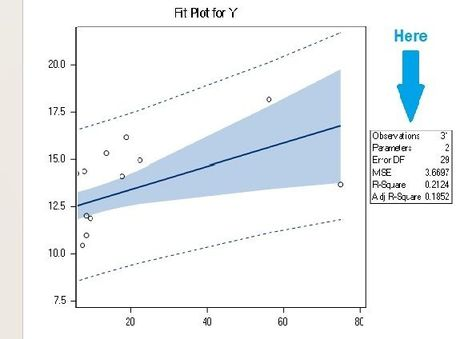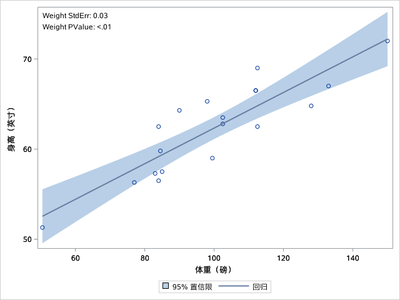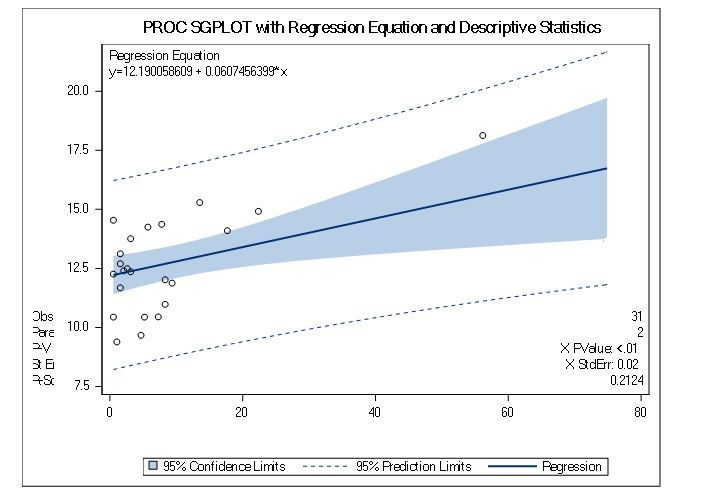## How to insert p-value in summary box of fit plots?

Does anyone know how to modify/edit the variables appearing in the fit plots' summary box (in the output)? I want to include the p-value and standard error.I am using:

PROC REG DATA=Test1 CORR;
MODEL Y=X / CLB P CLM;
RUN;

Thanks!

1 ACCEPTED SOLUTION

Accepted SolutionsKsharp
Super User

## Re: How to insert p-value in summary box of fit plots?

Save these statistics and Plot it by PROC SGPLOT.

```ods output ParameterEstimates=x;
PROC REG DATA=sashelp.class CORR ;
MODEL height=weight / CLB P CLM;
quit;

proc sql ;
select catx(' ',Variable,'StdErr:',put(StdErr,8.2)) into : StdErr from x
where Variable='Weight'
;
select catx(' ',Variable,'PValue:',put(Probt,pvalue8.2)) into : Probt from x
where Variable='Weight'
;
quit;
proc sgplot data=sashelp.class;
reg y=height x=weight/clm;
inset "&StdErr." "&Probt.";
run;```6 REPLIES 6Ksharp
Super User

## Re: How to insert p-value in summary box of fit plots?

Save these statistics and Plot it by PROC SGPLOT.

```ods output ParameterEstimates=x;
PROC REG DATA=sashelp.class CORR ;
MODEL height=weight / CLB P CLM;
quit;

proc sql ;
select catx(' ',Variable,'StdErr:',put(StdErr,8.2)) into : StdErr from x
where Variable='Weight'
;
select catx(' ',Variable,'PValue:',put(Probt,pvalue8.2)) into : Probt from x
where Variable='Weight'
;
quit;
proc sgplot data=sashelp.class;
reg y=height x=weight/clm;
inset "&StdErr." "&Probt.";
run;```## Re: How to insert p-value in summary box of fit plots?

Thank you so much, Ksharp.

I did try your code but it did not generate what I wanted. I found another one in this link https://support.sas.com/kb/65/202.html but the box with the summary is not shown correctly (as shown in the image). Any suggestions?Ksharp
Super User

## Re: How to insert p-value in summary box of fit plots?

Maybe there is not enough room for INSET.

yaxis min=-10;

## Re: How to insert p-value in summary box of fit plots?

Thank you so much, Ksharp.

I stopped using the software for several hours, and then when I tried again, it worked without putting your suggestion. However, now I get a warning that does not allow p-value and St Err to show in the summary box. As you can see in my previous graph, it worked (at least I could see the values), but now it does not. I wonder if it is a software issue. See the warning below. Any suggestion would be appreciated.

WARNING: Apparent symbolic reference PROBT not resolved.
229 "St Error"="&StdErr"
WARNING: Apparent symbolic reference STDERR not resolved.DanH_sas
SAS Super FREQ

## Re: How to insert p-value in summary box of fit plots?

You might want want to do a "proc print data=x; run;" to make sure those parameters are in your data set.Ksharp
Super User

## Re: How to insert p-value in summary box of fit plots?

As DanH said, make sure your macro variable generated correctly . To check it, open your dataset that generate these macro variable,and check if is there any obs/value to generate macro variable.
Discussion stats
• 6 replies
• 518 views
• 5 likes
• 3 in conversation